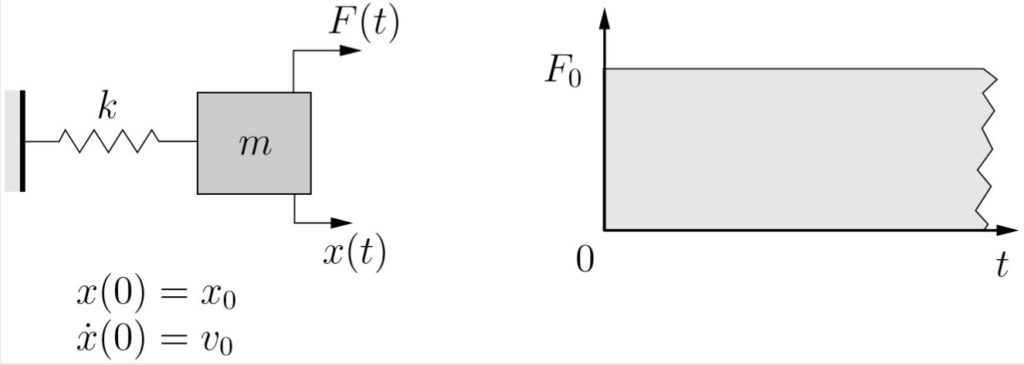### Transient Vibrations: Chapter 7 Examples

### Example 7-1

A mass, initially at rest, is subject to a step load as shown below. Determine the response of the system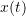for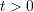. Use the method of convolution.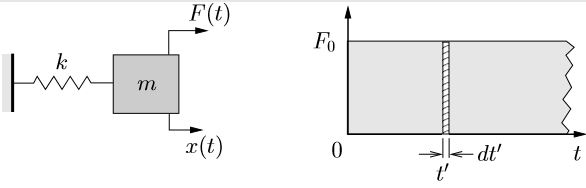### Example 7-2

A mass, initially at rest, is subject to a ramp load as shown below. Determine the response of the systemfor. Use the method of convolution.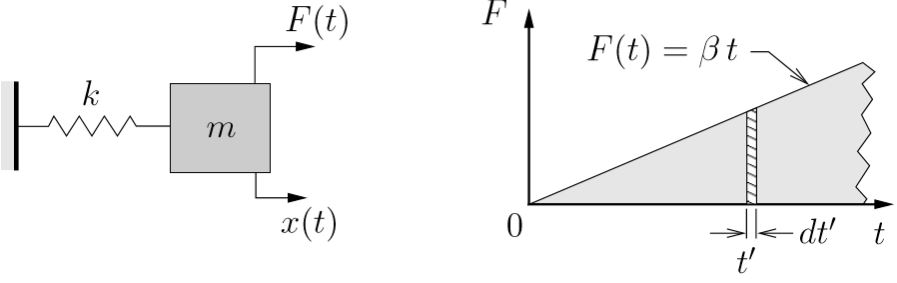### Example 7-3

A mass, initially at rest, is subject to a rectangular step load as shown below. Using the the method of convolution, determine the response of the systemfor

1.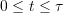,
2.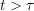.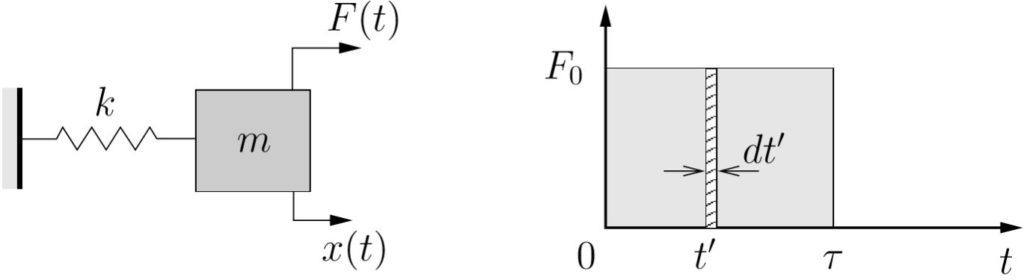### Example 7-4

A mass, initially at rest, is subject to a forcing function shown below. Using the the method of convolution, determine the response of the systemfor

1.,
2..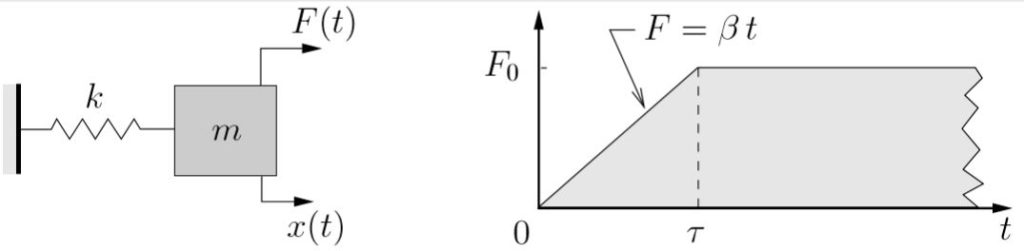### Example 7-5

A mass is initially moving such that at some time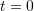the mass has position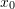and velocity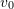. At that instant a step load is applied to the mass as shown below.
Determine the response of the systemforusing

1. Newton’s laws directly,
2. The method of convolution.